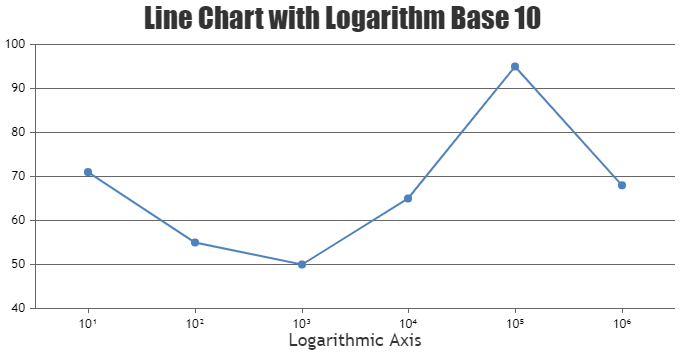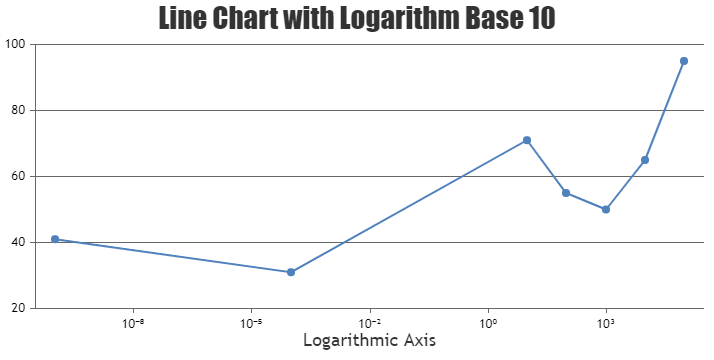Home Forums Feature Requests & Feedback logarithmic function

# logarithmic function

Viewing 9 posts - 1 through 9 (of 9 total)
• #29296

Dear Team,

I’d like to display values from -1000 tot 1000 in my graph on a logarithmic scale.
I understand that I cannot take the log of a negative number.

Is it possible to plot a negative value?

i can imagine something like
fictional_Log(100) = log(absolute(100))*1 = 2; (and see 100 as value in the graph within an logarithmic scale )
fictional_Log(-100) = log(absolute(-100))* -1 = -2; (and see -100 as value in the graph)

with kind regards,

Ron

#29306

Ron,

A logarithmic axis can only plot positive values. There simply is no way to put negative values or zero on a logarithmic axis.

Fundamental: If 10L = Z, then L is the logarithm (base 10) of Z. If L is a negative value, then Z is a positive fraction less than 1.0. If L is zero, then Z equals 1.0. If L is greater than 0, then Z is greater than 1.0. Note that there no value of L will result in a value of Z that is zero or negative. Logarithms are simply not defined for zero or negative numbers.

—-
Manoj Mohan
Team CanvasJS

#35697

How do i show the log values 10 to the power 3 in x- axis as like in the image? Log values

#35718

You can use unicode characters in labelFormatter to display power (subscript) values in the axis labels. Please check out the code snippet for the same.

``````var unicodeStringForSubscript = ["\u2070", "\u00B9", "\u00B2", "\u00B3", "\u2074", "\u2075", "\u2076", "\u2077", "\u2078", "\u2079"]
.
.
axisX:{
title: "Logarithmic Axis",
logarithmic: true,
logarithmBase: 10,
labelFormatter: function(e) {
return 10 + unicodeStringForSubscript[Math.ceil(Math.log(e.value)/Math.log(10))] ;
}
.
.
``````

Also, take a look at this JSFiddle for a complete working code.—-
Manoj Mohan
Team CanvasJS

#35721

Thanks a lot @manoj-mohan.

#35722

Does this handle both positive and negative values like 10^6 and 10^-6? it returns values as NaN when the values are in negative.

#35738

Previously shared code seems to work fine with positive values. The logic can be improved to make it work with negative values as shown in the below code-snippet

``````function getSubscriptString(value) {
var subscriptValue = Math.ceil(Math.log(value)/Math.log(10));

//Handling double digit numbers
if(subscriptValue > 9 || subscriptValue < -9) {
return (subscriptValue < -9 ? "\u207B" : "") + (Math.abs(subscriptValue)+"").split('').reduce(
function(prevVal,val) {
return prevVal + unicodeStringForSubscript[val];
}, "")
}

return (subscriptValue < 0 ? "\u207B": "") + unicodeStringForSubscript[Math.abs(subscriptValue)];
}
..
axisX:{
title: "Logarithmic Axis",
logarithmic: true,
logarithmBase: 10,
labelFormatter: function(e) {
return 10 + getSubscriptString(e.value);
}
}
..
``````

Also, check out this JSFiddle for complete working sample.—-
Manoj Mohan
Team CanvasJS

#35869

Hi Manoj,
Here I get the repeated values as of 10^5. How do i remove that values, if repeated?#35882

You can set the interval for axisX to overcome the issue of getting repeated values in x-axis.

If you are still facing the issue, can you kindly create JSFiddle reproducing the issue and share it with us so that we can understand your scenario better and help you out?

—-
Manoj Mohan
Team CanvasJS

Viewing 9 posts - 1 through 9 (of 9 total)

You must be logged in to reply to this topic.